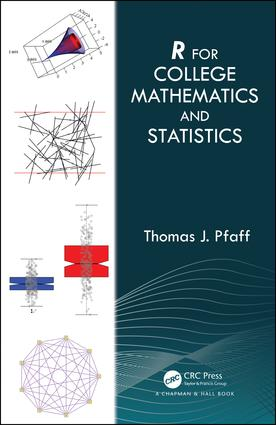# R For College Mathematics and Statistics

## 1st Edition

Chapman and Hall/CRC

326 pages | 99 B/W Illus.

##### Purchasing Options:\$ = USD
Hardback: 9780367196851
pub: 2019-04-23
Available for pre-order
\$99.95
x

FREE Standard Shipping!

### Description

R for College Mathematics and Statistics encourages the use of R in mathematics and statistics courses. Instructors are no longer limited to ``nice'' functions in calculus classes. They can require reports and homework with graphs. They can do simulations and experiments. R can be useful for student projects, for creating graphics for teaching, as well as for scholarly work. This book presents ways R, which is freely available, can enhance the teaching of mathematics and statistics.

R has the potential to help students learn mathematics due to the need for precision, understanding of symbols and functions, and the logical nature of code. Moreover, the text provides students the opportunity for experimenting with concepts in any mathematics course.

Features:

• Does not require previous experience with R
• Promotes the use of R in typical mathematics and statistics course work
• Organized by mathematics topics
• Utilizes an example-based approach
• Chapters are largely independent of each other

Getting Started

Importing Data into R

Functions and Their Graphs

A Piecewise-Defined Function. A Step Function. Polar Coordinates. Parametric Equations. Geometric Definition of a Parabola. Functions that Return a Function. Phythagorean Triples and a Checkerboard Plot.

Graphing

Graphing Functions. Scatter Plots. Dot, Pie, and Bar Charts. A look for loops. Boxplot with a Stripchart. Histogram.

Polynomials

Basic Polynomial Operations. The LCM and GCD of Polynomials. Illustrating Roots of a Degree–Three Polynomial. Creating Pascal’s Triangle with Polynomial Coefficients. Calculus with Polynomials. Taylor Polynomial of Sin(x). Legendre Polynomials.

Sequences, Series, and Limits

Sequences and Series. The Derivative as a Limit. Recursive Sequences.

Calculating Derivatives

Symbolic Differentiation. Finding Maximum, Minimum, and Inflection Points. Graphing a Function and Its Derivative. Graphing a Function with Tangent Lines. Shading the Normal Density Curve Outside the Inflection Points.

Riemann Sums and Integration

Riemann Boxes. Numerical Integration. Numerical Integration of Iterated Integrals. Area Between two Curves. Graphing an Antiderivative.

Planes, Surfaces, Rotations, and Solids

Interactive: Surface Plots. Interactive: Rotations around the x-axis. Interactive: Geometric Solids.

Curve Fitting

Exponential Fit. Polynomial Fit. Log Fit. Logistic Fit. Power Fit.

Simulation

A Coin Flip Simulation. An Elevator Problem. A Monty Hall Problem. Chuck-A-Luck. The Buffon Needle Problem. The Deadly Board Game.

The Central Limit Theorem and Z-test

A Central Limit Theorem Simulation. Z Test and Interval for One Mean. Z Test and Interval for Two Means.

The T-Test

T Test and Intervals for One and Two Means. Paired T-Test. Illustrating the Meaning of a Confidence Interval Simulation.

Testing Proportions

Tests and intervals for One and Two Proportions. Illustrating the Meaning of α Simulation.

Linear Regression

Multiple Linear Regression.

Nonparametric Statistical Tests

Wilcoxon Signed Rank Test for a Single Population. Wilcoxon Rank Sum Test for Independent Groups. Wilcoxon Signed Rank Test for Dependent Data. Spearman’s Rank Correlation Coefficient. Kruskal-Wallis one-way analysis of variance.

Miscellaneous Statistical Tests

One-way ANOVA. Stacking Data. Chi-Square Tests. Testing Standard Deviations.

Matrices

Eigenvalues, Eigenvectors and other Operations. Row Operations.

Differential Equations

Newton’s Law of Cooling. The Logistic Equation. Predator-Prey Mode.

Some Discrete Mathematics

Binomial Coefficients, Pascal’s Triangle, and a Little Number Theory. Set Theory. Venn Diagrams. Power Set, Cartesian Product, and Intervals. A Cantor Set Example. Graph Theory. Creating and Displaying Graphs. Random Graphs. Some Graph Invariants.

### About the Author

Thomas J. Pfaff is a professor of Mathematics at Ithaca College and served as the all college Honors Program director for three years. He was the PI on three-year NSF grant Multidisciplinary Sustainability Modules: Integrating STEM Courses. The scope of his publications range from traditional mathematics to applied mathematics, including the SABR newsletter, and sustainability to essays about higher education. His blog sustainbilitymath.org provides resources to incorporate sustainability ideas into mathematics courses and he is currently interested in using R for student projects in all courses.

### Subject Categories

##### BISAC Subject Codes/Headings:
BUS061000
BUSINESS & ECONOMICS / Statistics
MAT000000
MATHEMATICS / General